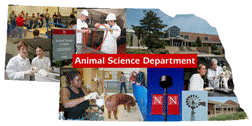## Animal Science Department## Faculty Papers and Publications in Animal Science

#### Date of this Version

March 2000

Published in J. Anim. Sci. 2000. 78:799–803.

#### Abstract

Crude fat content of longissimus (ribeye) muscle of beef cattle was predicted from a ratio of fat area (RFA) to area of ribeye muscle calculated from computer image analysis (CIA). Cross sections of 64 ribeyes taken from the 6–7th rib from cattle at experiment station A and cross sections of 94 ribeyes taken from the 6–7th rib from cattle at Experiment Station B were used in this study. Slices (1 to 1.5 cm in thickness) of just the Longissimus dorsi were homogenized and sampled for chemical estimation of crude fat content using petroleum ether. Crude fat content as determined from chemical analysis was used as the true estimate of fat content. A CCD (charge-coupled device) camera was used as the input device at Experiment Station A, and a single-lens reflex camera was used at Experiment Station B to photograph ribeyes for CIA. The contour comparison method, which assigns a threshold value for each marbling particle, was used to obtain accurate binarization in this study. Minimum and maximum of chemical measurements of crude fat were 2.1 and 39.8%, and for CIA calculation of the RFA were 6.1 and 56.8%, respectively. This range covered almost the complete range of the beef marbling standard used in carcass grading in Japan. The equation for the regression of the crude fat content (Y) on RFA (X) calculated from CIA for all of the data was Y = .793X − 3.04 with r2 = .96. Regression equations for prediction of crude fat percentage from RFA taking into consideration the effect of experiment station were Y = .741X − 2.22 with r2 = .91 for Experiment Station A, and Y = .782X − 2.54 with r2 = .91 for Experiment Station B. Analysis of covariance showed that the effects of experiment stations on intercepts and slopes were not significant (P > .10). The ranges of differences between actual and predicted crude fat content from the prediction equation that was calculated without consideration of the effect of station were − 6.4 to 4.0%. CIA of cross sections of the ribeye muscle seems to have potential for prediction of crude fat content.

COinS